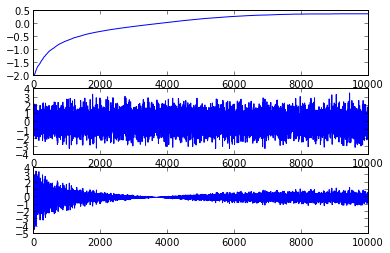Goal: choose a multiplier $a$ s.t. the power of the amplified signal is approximiately equal to some specified target power, $d^2$. We take the input signal, $r(t)$ and multiply it to get $s(t) = a\cdot r(t)$. Specifically,

$d^2 = E((a \cdot r(t))^2)$

A bit of finagling, and we get

$a^2 \approx \frac{d^2}{avg[r^2(kT)]}$

We use the boring old average for this avg function, ie the arithmetic mean of the samples. The arithmetic mean is a kind of janky lowpass filter, so maybe it would be better to use one of those instead, but, hey, here we are.

We now want to make this adaptive, so it can react to changes in the environment. In the text, the following is carefully derived (p112):

$J_{Least Squares} = . a[k+1] = a[k] - avg { (s2[k]-d2) } | _{k=NN-j}$

There is also a note that one should avoid letting $a[k]$ get too close to zero, which will clearly cause the rightmost fraction to grow rather large, which may blow out the fitting mechanism.

They also derive a different function:

$J_{Naive} = a[k+1] = a[k] - avg{sgn(a[k])(s2[k]-d2)}$

In :
# agcgrad.m: Minimize J(a) = avg{|a|((1/3)a*a*r*r-ds)} by choice of a

n = 10000
vr = 1.0
r = sqrt(vr)*random.normal(0,1,n)

ds = 0.15 # desired output power, 0.15 = 0.39^2
mu = 0.001 # stepsize
lenavg = 10 # j in above

# Initialize our two output arrays
a = zeros(n)
s = zeros(n)

for k in range(0,lenavg):
a[k] = 1
s[k] = r[k]

for k in range(lenavg,n):
a_kmo = a[k-1]
s_window = s[k-lenavg:k]

ak = a_kmo - mu * mean( sign(a_kmo)*s_window*s_window - ds)

a[k] = ak
s[k] = ak * r[k]

fig = figure()
ax1.plot(a)
ax2.plot(r)
ax3.plot(s)

a[n-1]

Out:
0.39086392457251146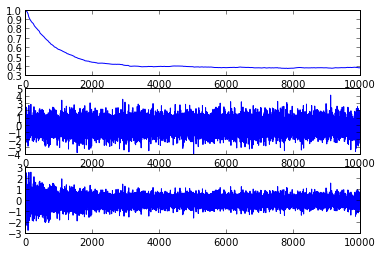In :
def genAGC(ds, mu, lenavg):
"""Returns a function that implements AGC for the given params
ds = 0.15 # desired output power, 0.15 = 0.39^2
mu = 0.001 # stepsize
lenavg = 10 # j in above
"""

def retval(r):

# Initialize our two output arrays
a = zeros(n)
s = zeros(n)

for k in range(0,lenavg):
a[k] = 1
s[k] = r[k]

for k in range(lenavg,n):
a_kmo = a[k-1]
s_window = s[k-lenavg:k]

ak = a_kmo - mu * mean( sign(a_kmo)*s_window*s_window - ds)

a[k] = ak
s[k] = ak * r[k]

return (a,s)

return retval

def runOne(vr, mu, lenavg):
n = 10000
vr = 1.0
r = sqrt(vr)*random.normal(0,1,n)

agc = genAGC(0.15, mu, lenavg)
(a,s) = agc(r)

# Draw plots
fig = figure()

ax1.plot(a)

ax2.plot(r)

ax3.plot(s)

# Look at how the convergence is doing after it's settled in a bit
ax4.plot(diff(a)[2000:n-1])

*6.17* Use agcgrad.m to investigate the AGC algorithm. a. What range of stepsize mu works? Can the stepsize be too small? Can the stepsize be too large? b. How does mu affect convergence? c. How does the variance of the input affect the convergent value of a? d. What range of lenavg works? Can lenavg be too small? Too large? e. How does lenavg affect the convergence rate?
In :
# a, b: Look at the behavior of convergence with varying mu values
runOne(1.0, 0.00001, 10)
runOne(1.0, 0.001, 10)
runOne(1.0, 0.01, 10)
runOne(1.0, 0.1, 10)
runOne(1.0, 1.0, 10)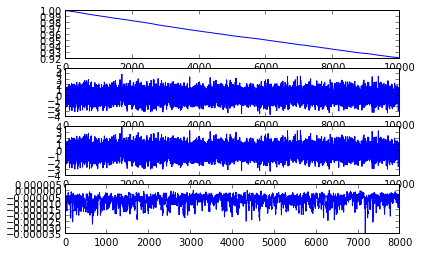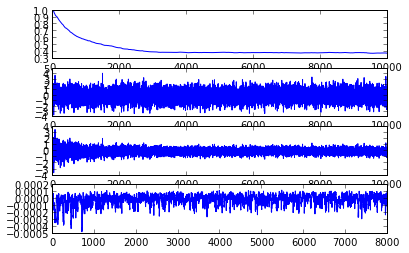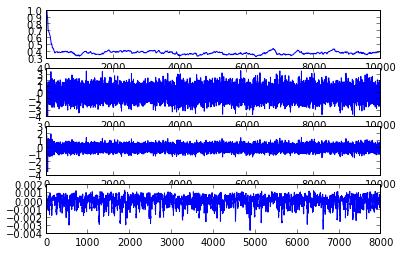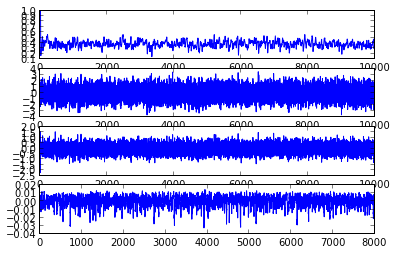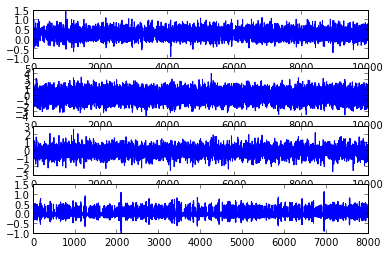This is what we'd expect: too small of $\mu$ and it never converges, too large of $\mu$ and it spends all its time bouncing around wildly.

In :
# c) Look at the behavior of convergence with varying variance of input signal
runOne(1.0, 0.001, 10)
runOne(0.1, 0.001, 10)
runOne(10.0, 0.001, 10)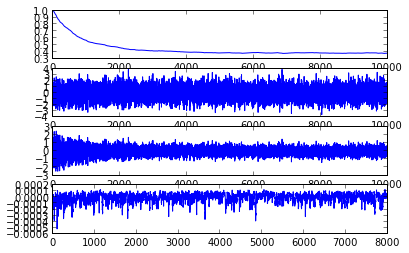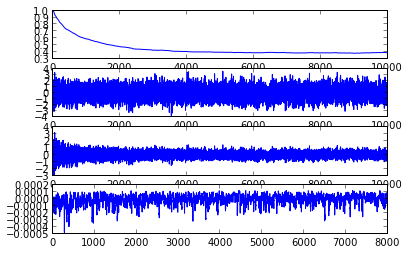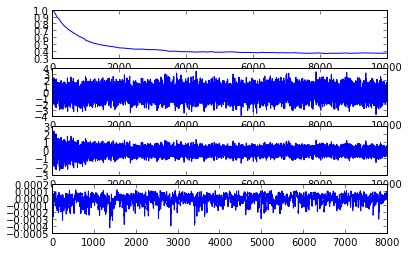This is actually really interesting. We see that they converge at pretty much exactly the same rate. This makes sense, since the derivative of the next $a$ value with respect to the magnitude of $r$ is linear in $r$. It's still neat to see.

In :
# d,e: how does lenavg affect convergence?

runOne(1.0, 0.001, 10)
runOne(1.0, 0.001, 3)
runOne(1.0, 0.001, 20)
runOne(1.0, 0.001, 50)
runOne(1.0, 0.001, 100)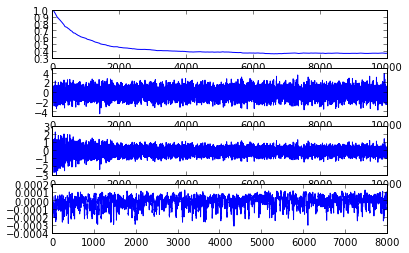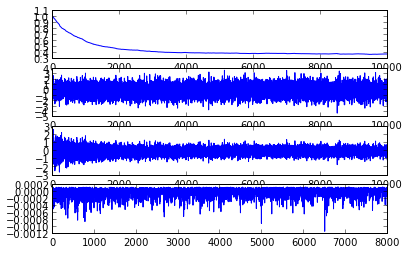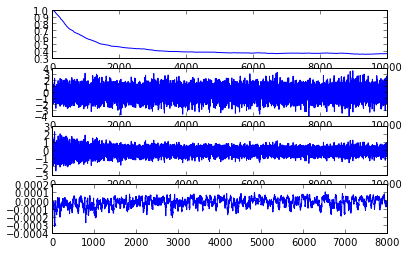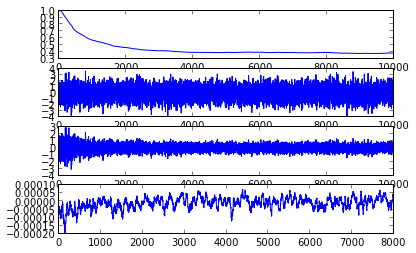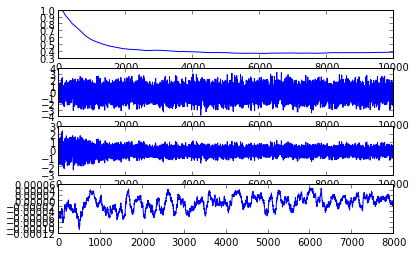This is another "duh" graph, much like the one for $\mu$ above. As the averaging length gets longer, the algorithm gets less twitchy.

6.20. Try initailizing a(1)=-2. What minimum does it find? What happens to the data?

In :
# agcgrad.m: Minimize J(a) = avg{|a|((1/3)a*a*r*r-ds)} by choice of a

n = 10000
vr = 1.0
r = sqrt(vr)*random.normal(0,1,n)

ds = 0.15 # desired output power, 0.15 = 0.39^2
mu = 0.001 # stepsize
lenavg = 10 # j in above

# Initialize our two output arrays
a = zeros(n)
s = zeros(n)

for k in range(0,lenavg):
a[k] = -2
s[k] = r[k]

for k in range(lenavg,n):
a_kmo = a[k-1]
s_window = s[k-lenavg:k]

ak = a_kmo - mu * mean( sign(a_kmo)*s_window*s_window - ds)

a[k] = ak
s[k] = ak * r[k]

fig = figure()

0.37879772727274846